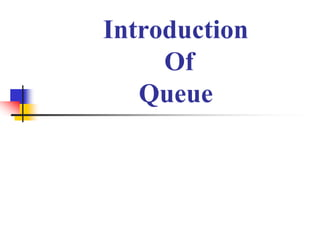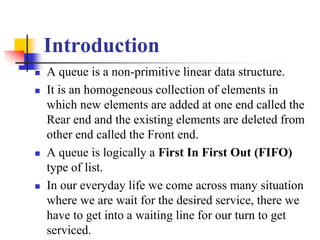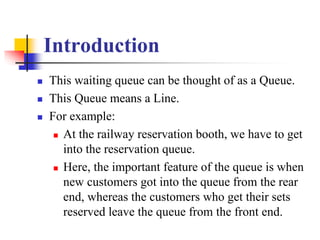Se está descargando tu SlideShare. ×

# Data structure.ppt

Anuncio
Anuncio
Anuncio
Anuncio
Anuncio
Anuncio
Anuncio
Anuncio
Anuncio
Anuncio
AnuncioCargando en…3
×

1 de 26 Anuncio

# Data structure.ppt

Hi everyone i hope you will be fine plz support me i hope you well statisfied with this slide and course.

Hi everyone i hope you will be fine plz support me i hope you well statisfied with this slide and course.

Anuncio
Anuncio

### Data structure.ppt

1. 1. Introduction Of Queue
2. 2. Introduction  A queue is a non-primitive linear data structure.  It is an homogeneous collection of elements in which new elements are added at one end called the Rear end and the existing elements are deleted from other end called the Front end.  A queue is logically a First In First Out (FIFO) type of list.  In our everyday life we come across many situation where we are wait for the desired service, there we have to get into a waiting line for our turn to get serviced.
3. 3. Introduction  This waiting queue can be thought of as a Queue.  This Queue means a Line.  For example:  At the railway reservation booth, we have to get into the reservation queue.  Here, the important feature of the queue is when new customers got into the queue from the rear end, whereas the customers who get their sets reserved leave the queue from the front end.
4. 4. Introduction  It means the customers are serviced in the order in which they arrive the service center.  This same characteristics apply to our Queue.  The next slide figures show queue graphically during insertion and deletion operations.
5. 5. Introduction  The following figures show queue graphically during insertion operation's=Front & R=Rear 10 20 10 (a) Empty Queue F=-1 & R=-1 0 1 2 3 4 (b) One element inserted in queue F=0 & R=0 0 1 2 3 4 0 1 2 3 4 (b) Second element inserted in queue F=0 & R=1 F R F R F R
6. 6. Introduction  The following figures show queue graphically during deletion operation's=Front & R=Rear 10 20 20 (a) Empty Queue F=-1 & R=-1 0 1 2 3 4 (b) One element inserted in queue F=1 & R=1 0 1 2 3 4 0 1 2 3 4 (b) Second element inserted in queue F=0 & R=1 F R F R F R
7. 7. Queue Implementation  There are two ways to implement a Queue:  Static implementation (using array)  Dynamic implementation (using Pointer/Linked list)
8. 8. Static Implementation (using array)  If queue is implemented using arrays, we must be sure about the exact number of elements .  We want to store in the queue, because we have to declare the size of the array at design time or before the processing starts.  In this case, the beginning of the array will become the front for the queue and the last location of the array will act as rear for the queue.
9. 9. Static Implementation (using array)  The following relation gives the total number of elements present in the queue, when implemented using arrays: Total no of elem.=front-rear+1  Also note that if rear<front then there will be no element in the queue or queue is always be empty.
10. 10. Dynamic implementation (using Pointer/Linked list)  Implementing queues using pointers, the main disadvantage is that a node in a linked representation occupies more memory space then a corresponding element in the array representation.
11. 11. Types of Queue  There are many different way to implement a Queue:  Simple Queue/Linear Queue  Circular Queue  Double Ended Queue (Deque)  Priority Queue
12. 12. Simple Queue  In the simple queue, the beginning of the array will become the front for the queue and the last location of the array will act as rear for the queue.  The following relation gives the total number of elements present in the queue, when implemented using arrays: Total no of elem.=front-rear+1
13. 13. Simple Queue  Also note that if rear<front then there will be no element in the queue or queue is always be empty.  Bellow show a figure a empty simple queue Q which can accommodate five elements. Q Q Q Q Q Way of deletion Way of insertion Fig: Simple Queue
14. 14. Limitation of Simple Queue  There are certain problems associated with a simple queue when queue is implemented using array.  Consider an example of a simple queue Q, which is initially empty.  We can only five elements insertion in queue.  If we attempt to add more elements, the elements can’t be inserted because in a simple queue rear increment up to size-1.
15. 15. Limitation of Simple Queue  At that time our program will flash the message “Queue is full”.  But if the queue is too large of say 5000 elements it will be a tedious job to do and time consuming too.  To remove this problem we use circular queue.
16. 16. Circular Queue  A circular queue is one in which the insertion of a new element is done at the very first location of the queue if the last location of the queue is full.  In other words if we have a queue Q of say n elements, then after inserting an element last (n-1th) location of the array the next elements will be inserted at the very first location (0) of the array.  It is possible to insert new elements, if and only if those location (slots) are empty.
17. 17. Circular Queue  We can say that a circular queue is one in which the first element comes just after the last element.  It can be viewed as a mesh or loop of wire, in which the two ends of the wire are connected together.  A circular queue overcomes the problem of unutilized space in linear queues implemented as arrays.  A circular queue also have a Front and Rear to keep the track of the elements to be deleted and inserted and therefore to maintain the unique characteristic of the queue.
18. 18. Circular Queue  Bellow show a figure a empty circular queue Q which can accommodate five elements. ` Q Q Q Q Q Fig: Circular Queue
19. 19. Double Ended Queue (Deque)  It is also a homogeneous list of elements in which insertion and deletion operations are performed from both the ends.  That is, we can insert elements from the rear end or from the front ends.  Hence it is called double-ended queue. It is commonly referred as a Deque.  There are two types of Deque. These two types are due to the restrictions put to perform either the insertions or deletions only at one end.
20. 20. Double Ended Queue (Deque)  There are:  Input-restricted Deque.  Output-restricted Deque.  Bellow show a figure a empty Deque Q which can accommodate five elements. Q Q Q Q Q deletion deletion insertion insertion Fig: A Deque
21. 21. Double Ended Queue (Deque)  There are:  Input-restricted Deque: An input restricted Deque restricts the insertion of the elements at one end only, the deletion of elements can be done at both the end of a queue. 10 20 30 40 50 Q Q Q Q Q deletion deletion insertion Fig: A representation of an input-restricted Deque F R
22. 22. Double Ended Queue (Deque)  There are:  Output-restricted Deque: on the contrary, an Output-restricted Deque, restricts the deletion of elements at one end only, and allows insertion to be done at both the ends of a Deque. 10 20 30 40 50 Q Q Q Q Q insertion deletion insertion Fig: A representation of an Output-restricted Deque F R
23. 23. Double Ended Queue (Deque)  The programs for input-restricted Deque and output-restricted Deque would be similar to the previous program of Deque except for a small difference.  The program for the input-restricted Deque would not contain the function addqatbeg().  Similarly the program for the output-restricted Deque would not contain the function delatbeg().
24. 24. Priority Queue  A priority queue is a collection of elements where the elements are stored according to their priority levels.  The order in which the elements should get added or removed is decided by the priority or the element.  Following rules are applied to maintain a priority queue.  The element with a higher priority is processes before any element of lower priority.
25. 25. Priority Queue  If there are elements with same priority, then the element added first in the queue would get processed  Here, smallest number that is most highest priority and greater number that is less priority.  Priority queues are used for implementing job scheduling by the operating system.  Where jobs with higher priorities are to be processed first.  Another application of priority queue is simulation systems where priority corresponds to event times.
26. 26. Applications of Queues  Round robin technique for processor scheduling is implemented using queues.  All types of customer service (like railway ticket reservation) center software’s are designed using queues to store customers information.  Printer server routines are designed using queues. A number of users share a printer using printer server the printer server then spools all the jobs from all the users, to the server’s hard disk in a queue. From here jobs are printed one-by-one according to their number in the queue.